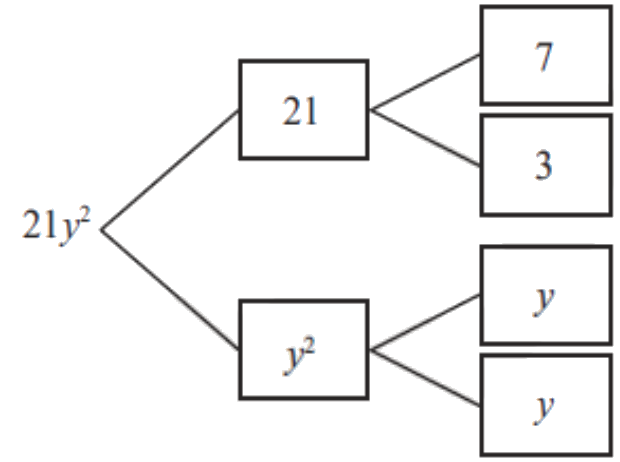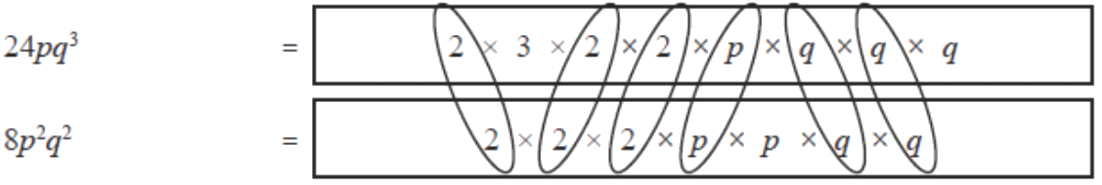# Algebra - Factorisation

Go back to  'Algebra Basics'

## Introduction to Factorization of Algebraic Expressions

The most common question asked by students when they are faced with algebra problems is how does it apply to their everyday lives? The fact of the matter is that algebra is abstraction and largely a mathematical tool. However, the habit of approaching problems systematically and methodically is what essentially translates to problem solving abilities in real life.

## What are factors? What is factorisation?

Think of factors as the ingredients of something.

$$1,\,2,\,3,\,4,\,6$$  and $$12$$  are the factors of $$12.$$ That means you can create $$12$$ using some of these ingredients.

\begin{align}3 \times 4 = 12 \\ 2 \times 6 = 12 \end{align}

Similarly, algebraic terms also have factors. These are the ingredients that make that term. So, the term $$6x^2$$ can be split into its ingredients

$$6x^2 = 2 \times 3 \times x \times x$$

 The process of finding components (or factors) which when multiplied give the original expression is called factorisation.

## The factorisation of algebraic expressions

Example 1

Consider the expression $$4x^2 + 8.$$

• There are two terms. Do they have any factor common?
• The second term “$$8$$” can be re-written as $$(4 \times 2).$$
• So, $$4$$ is a common factor in the two terms.
• We can now factorise the expression:
$$4x^2 + 8 = 4 (x^2 + 2)$$

Verify: Always multiply the factorised expression and see if you get the original expression back.

Example 2

Consider $$4y^3 + 2y^2.$$

• Again, we have two terms. Do they have any factor common?
• Both terms have $$2$$ as a common factor. But that’s not all. Even $$y^2$$ is common.
• So, the highest common factor is $$2y^2.$$ That is, the second term is also a factor of the first.
• We can now factorise the expression:
\begin{align}4y^3 + 2y^2 = 2y^2 (2y + 1) \end{align}

Verify: Always multiply the factorised expression and see if you get the original expression back.

Example 3

Consider $$z^2 + 5z + 2z + 10.$$

• Here we have four terms (actually $$3,$$ because two terms are like terms. But let’s ignore that for a moment). Do they have any factor common?
• Looking at the $$4$$ terms at once we see there are no common factors to all four.
• However, observing carefully shows a pattern: $$5z$$ and $$10$$ have $$5$$ as a common factor. And $$z^2$$ and $$2z$$ have $$z$$ as a common factor. And when we factorise these common terms, we are left with $$(z + 2)$$ in both cases.
$$z^2 + 5z + 2z + 10 = z (z + 2) + 5\,(z + 2)$$
• Observe that we are down to two terms. And these two terms have $$(z + 5)$$ common. So, we get:
$$(z + 2)\,(z + 5)$$

Verify: Always multiply the factorised expression and see if you get the original expression back.

Example 4

Consider $$x^2 + 8x + 16$$

• Just by observing the terms we see that there is no factor common to all three.
• But in some cases, we can use algebraic identities to help us out.
• Did you notice the constant term, $$16,$$ is a perfect square? $$16 = 42.$$
• This makes our expression similar to the identity $$a^2 + 2ab + b^2 = (a + b)^2.$$
• Here $$a = x$$ and $$b = 4.$$
• Using this identity to factorise we get:
\begin{align} x^2 + 8𝑥 + 16 & = x^2 + 2 (4x) + 42 \\ & = (x + 4)^2 \end{align}

Example 5

Consider $$9y^2 - 25$$

• There seems to be no factor common to these two terms on first glance.
• But we see that both terms are perfect squares with a subtraction sign between them.
• This clue should make us think of the identity: $$a^2 - b^2 = (a + b) (a - b)$$
• Using this identity to factorise we get:
\begin{align} 9y^2 - 25 &= (3y)^2 - 5^2 \\ &= (3y + 5) (3y - 5)\end{align}

Remember the most important identities which will help you factorise expressions quickly

## List of the most important and useful identities

• $$(a + b)^2 = a^2 + 2ab + b^2$$
• $$(a - b)^2 = a^2 - 2ab + b^2$$
• $$(a + b)(a - b) = a^2 - b^2$$
• $$(x + a) (x+ b) = x^2 + x(a+b) + ab$$

## Tips and Tricks to Factorise

• Finding factors of a monomial is best visualised as finding the prime factors of a whole number:So, $$21y^2 = 7 \times 3 \times y \times y$$

• Factorising algebraic expressions is the same as finding the HCF of two numbers. So visualise the process as if you were finding the HCF of two whole numbers:
E.g. To find the common factors of $$24pq^3$$ and $$8p^2q^2$$ use this trick:Thus the common factor is $$8pq^2.$$

• Keep in mind that when factorising sometimes the common factor will have constants as well as variables. It is easy to miss one of these and end up with an incompletely factored expression.
E.g. $$(6ab - 9ab^2)$$ may get factored as $$3a(2b - 3b^2)$$ instead of $$3ab(2 - 3b).$$
Always double-check after factorising to see if there is another possible common factor that can be pulled out.

• When factorising (monic) quadratic expressions, a common trick is to split the middle term such that the coefficients of the split terms add up to the original coefficient and when multiplied gives you the constant term.